# Test Prep Plan - Take a practice test

Take this practice test to check your existing knowledge of the course material. We'll review your answers and create a Test Prep Plan for you based on your results.
How Test Prep Plans work
1
2Based on your results, we'll create a customized Test Prep Plan just for you!
3Study smarter
Study more effectively: skip concepts you already know and focus on what you still need to learn.

# NES Math: Rate of Change Chapter Exam

Exam Instructions:

Choose your answers to the questions and click 'Next' to see the next set of questions. You can skip questions if you would like and come back to them later with the yellow "Go To First Skipped Question" button. When you have completed the practice exam, a green submit button will appear. Click it to see your results. Good luck!

### Page 1

#### Question 1 1. Where is y differentiable?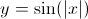#### Question 2 2. Consider the graph below. Between x=C and x=-C, for any value of C, will there be a point where the instantaneous rate of change is 0?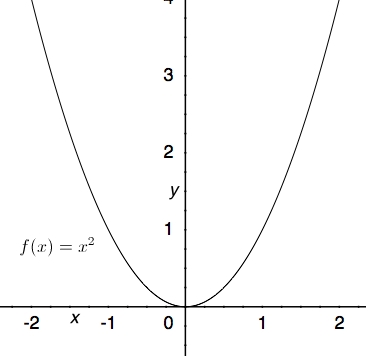#### Question 3 3. Consider the function f(x) given below. According to Rolle's theorem, for how many values of x is the instantaneous rate of change equal to 0?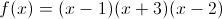#### Question 4 4. What is the derivative of the following?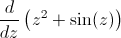#### Question 5 5. The derivative of f(x) is equal to 0 at how many points on the graph below?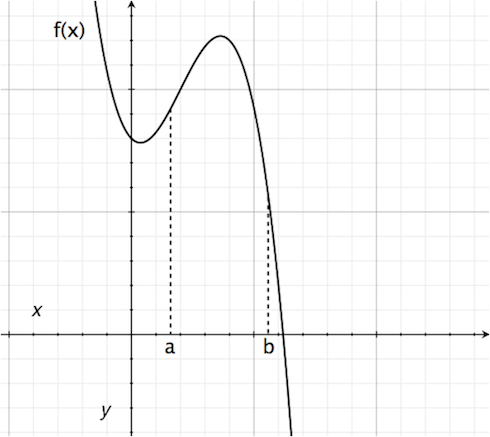### Page 2

#### Question 6 6. At how many points on the graph below is the derivative of f(x)=0?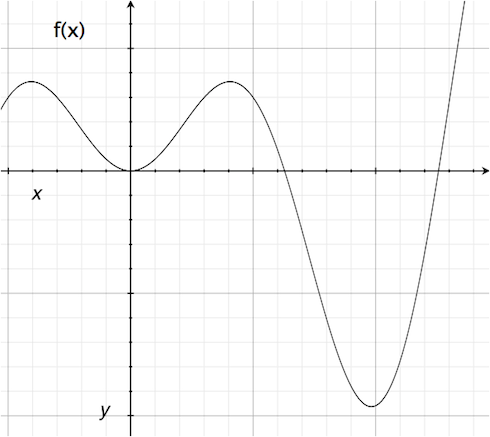#### Question 8 8. What is the value of f(x) at the red circle?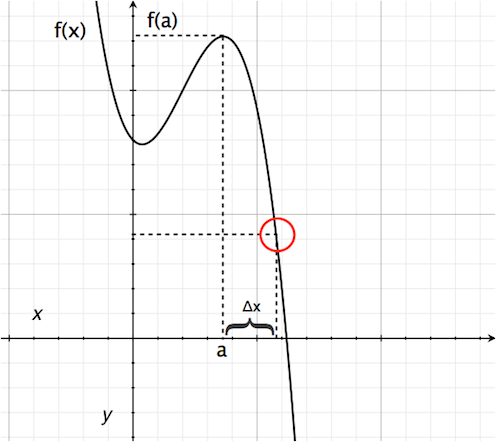#### Question 10 10. What is the average rate of change for the interval with endpoints A and B?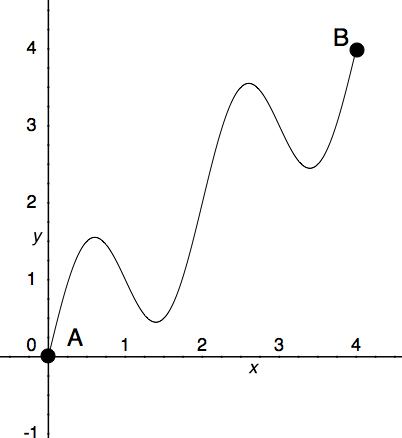### Page 3

#### Question 11 11. Where is f(x) differentiable?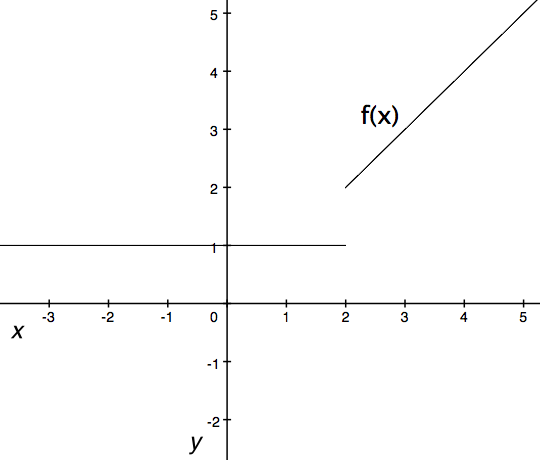#### Question 12 12. Where t= time, what is the derivative of f(t)?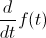#### Question 14 14. Which point on the following graph satisfies the mean value theorem between x = 0 and x = 3?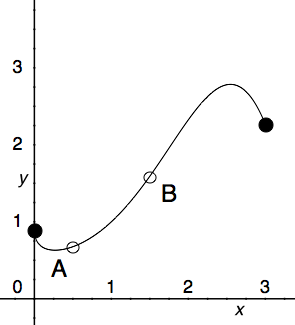#### Question 15 15. Consider the function f(x) given below. According to Rolle's theorem, between x=0 and x=pi, for how many values of x is the instantaneous rate of change equal to 0?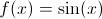### Page 4

#### Question 16 16. What is the rate of change at the point E in the graph below (for y in relation to x)?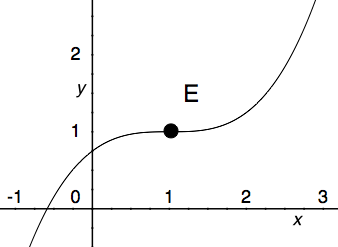#### Question 17 17. Consider the graph and question below..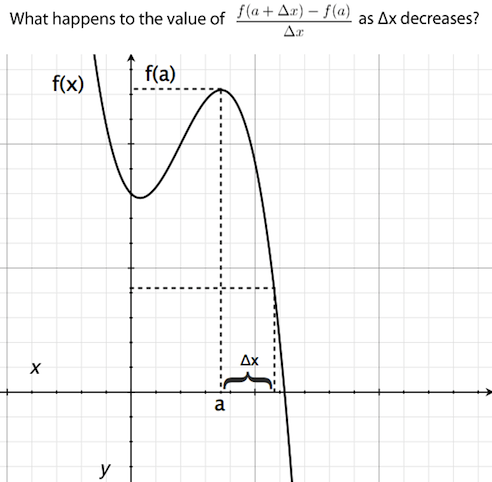#### Question 18 18. What is the rate of change at the point A in the graph below (for y in relation to x)?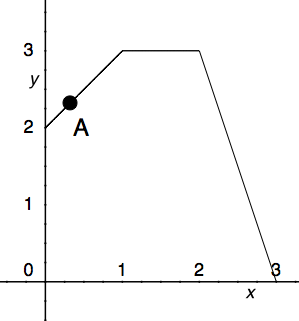#### Question 19 19. What is the rate of change at the point A in the graph below (for y in relation to x)?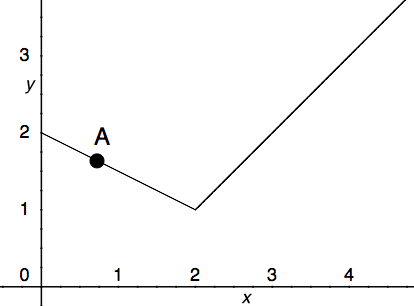#### Question 20 20. Where is f(x) differentiable?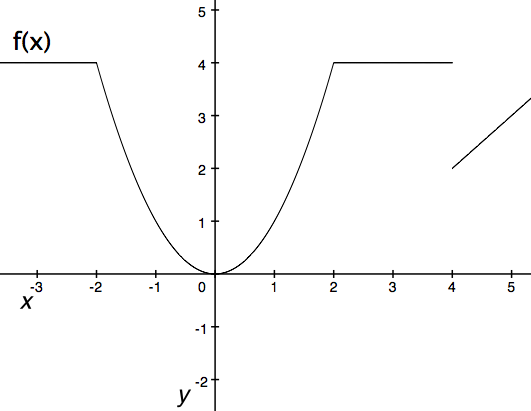### Page 5

#### Question 22 22. What is the rate of change at the point B in the graph below (for y in relation to x)?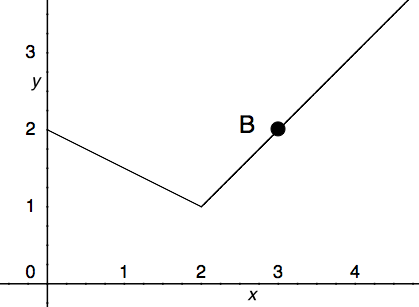#### Question 23 23. Where is f(x) differentiable?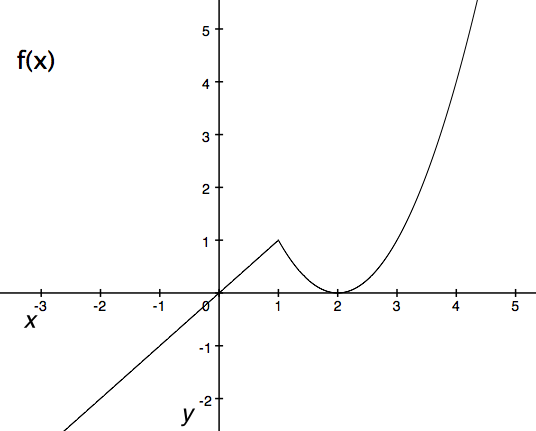#### Question 24 24. According to the mean value theorem, at how many points will the instantaneous rate of change equal the average rate of change between x = 1 and x = 3 on the following graph?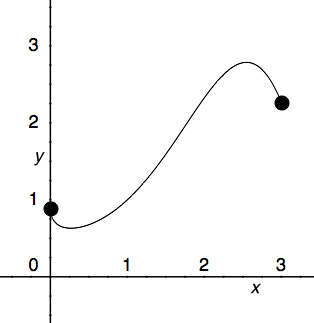#### Question 25 25. Where is f(x) differentiable?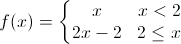### Page 6

#### Question 28 28. What is the rate of change at the point B in the graph below (for y in relation to x)? (In other words, what is the slope of the line from x = 2 to x = 3?)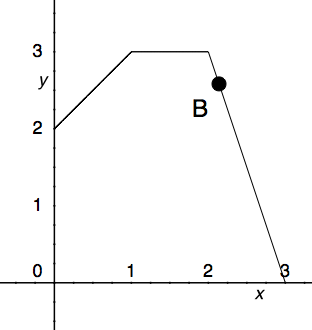#### Question 29 29. Which is greater, the derivative at a or the derivative at b?#### Question 30 30. What is the approximate instantaneous rate of change at point C?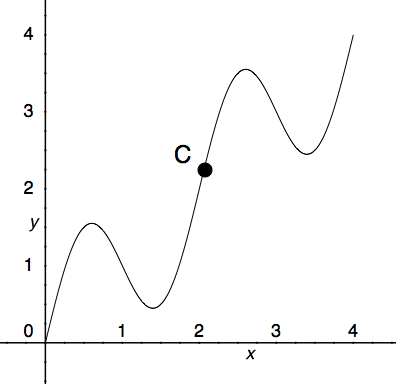#### NES Math: Rate of Change Chapter Exam Instructions

Choose your answers to the questions and click 'Next' to see the next set of questions. You can skip questions if you would like and come back to them later with the yellow "Go To First Skipped Question" button. When you have completed the practice exam, a green submit button will appear. Click it to see your results. Good luck!

Support